# The Dilution Effect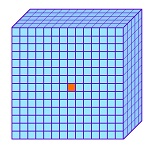Nov79.com

A major error of global warming is defying the fact that each carbon dioxide molecule is surrounded by 2,500 air molecules and has no way to spread the heat around. This dilution divides the CO2 temperature by 2,500 for the average temperature, which is no heating at all.

To heat the air 1°C average, each CO2 molecule would have to be 2,500°C—an impossibility. At 400 parts per million in the atmosphere, CO2 is too dilute to heat the surrounding air.

Conducting heat to nearby molecules by bumping increases the number of molecules radiating away the added heat, which prevents very many molecules from picking up additional heat.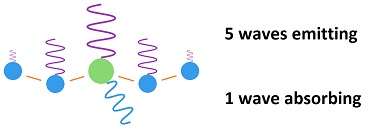If 5 air molecules radiate away the added energy, while one CO2 molecule absorbs, the added energy is gone and the other 2,495 air molecules surrounding each CO2 molecule are not affected. There cannot be a significant temperature increase in the atmosphere when 2,495 air molecules surrounding each CO2 molecule are unaffected by the process.

Absorbed radiation by CO2 would be re-emitted in 83 femto seconds, which means it does not significant increase in temperature. The time is calculated from the emitted wavelength. All matter emits infrared radiation due to the vibration of molecules, which is called black body radiation.

The average wavelength for radiation emitted from the earth is 25 microns, which means 83 femto seconds per wave. While one wave is being absorbed at 15 microns, another wave is being emitted at 25 microns. The emitted wave is stronger than the absorbed wave, because the absobed wave is limited to 8% of the black body radiation, which is called fingerprint radiation.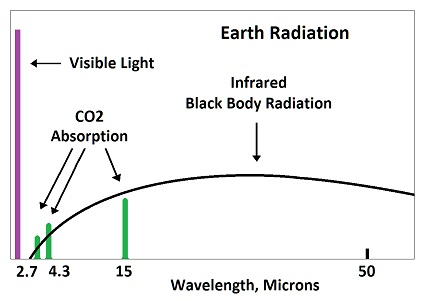The average wavelength of radiation emitted by all molecules including CO2 at near-surface temperatures would be around 25 microns, which is about the center of the graph above. In emitting radiation at 25 microns, there are 83 femto seconds for each initial bump. (frequency equals velocity over wavelength. Time equals inverse of frequency) (The speed of light is 3×108 meters per second.) (3×108 ÷ 25×10-6 = 12×1012, inverse = 83×10-15).

The sequence of events is this: During half of a vibration, half of the energy of a wave is emitted. Then the CO2 molecule bumps into a nearby molecule, which is mostly nitrogen. One half of its energy is given up to the molecule which it bumps into.

This adds up to one half of one half, which is one fourth of the energy of one wave, which is passed onto the nearby molecule, while three fourths is radiated away. The bumped molecule does the same thing and passes on one fourth of its acquired energy to the molecule it bumps while radiating away three fourths.

The net effect is that three fourths of the energy picked up by the CO2 is radiated away in 83 femto seconds, while one fourth is passed onto a nearby molecule, which radiates away three fourths of its acquired in 83 femto seconds. The second molecule bumped gets no more than a trace of the original energy acquired by the CO2 molecule.

On top of that, saturation only allows a small percent of the CO2 to be adding heat. No one can say what percent, but the the figure 5% used to be used—perhaps being ignored later due to being ridiculously high. That number would require increasing the dilution by another factor of 20, which is 50,000°C for each CO2 molecule for an average of 1°C for the entire atmosphere.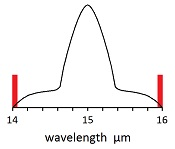Actually, one thousandths of the CO2 would still saturate, which means the 2,500 dilution would be 2.5 million dilution at the break-point for saturation. The reason is because saturation occurs in 10 meters for most of the CO2. Dividing 10 meters by 1,000 is 10 kilometers, which is the edge of the troposphere.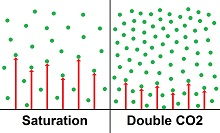Climatologists admit that the temperature of the CO2 molecules in the air is about the same as the temperature of the rest of the air, which would have to be the case. Yet each CO2 molecule is supposed to heat the surrounding 2,500 air molecules to 1°C upon doubling the amount of CO2 in the air.

Doing this would require CO2 to be a cold conduit for heat. There is no such thing as a cold conduit for heat. Temperature conductivity coefficients show that a very significant temperature gradient is needed to conduct heat.

If heat were passing through CO2 at almost the same temperature as the atmosphere and heating the rest of the air molecules, the heat would need to be accumulating in the air. Accumulation of heat cannot occur in the atmosphere, as absorbed radiation is approximately re-emitted in 83 femto seconds.